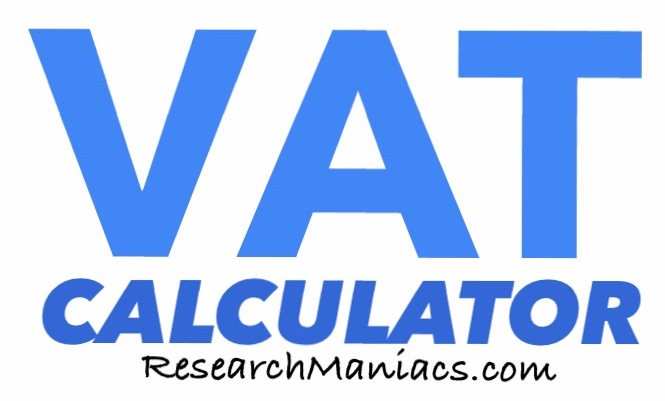What is £125 plus VAT?Use this information if you are buying something that costs £125 without VAT, and you want to know how much it will cost with Value-Added Tax (VAT).

£125 plus VAT is shown in green below:

£150.00

The amount in green above is calculated using the VAT rate in the box below. You can adjust the VAT rate and recalculate to adjust to your situation.

The amounts on this page are listed in pound sterling, but you could substitute the £ for another currency and the answer would still be correct.

We used the following formula to calculate £125 plus VAT:

T = A + ((A * V)/100)

In the formula above, V is the VAT rate and A is £125 and T is the answer.

VAT Calculator
Enter another amount below to see what it will cost with VAT.

What is £125.95 plus VAT?
Go here for the next amount on our list of VAT calculations.